# Expected maxima of a Brownian Motion- Does my stock trading strategy work?

I recently realized that I have a certain habit when it comes to stock trading even though I used to tell myself that “every trading strategy is useless because the stock market is just random”. If I buy a share I usually set the limit just a little below the actual price while if i sell I place the limit above. The question we want to answer today is if such a trading strategy is reasonable and if there is an optimal limit to maximize the expected profit.

More formally, letbe the stock price at timeand letbe a limit set for a certain order, then the order will be fulfilled iffor some. In the following we will investigate how to set a best price if an order should be processed within a given time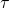(1)To answer the questions we have deduct a model based on some assumptions. A popular start is to think about a stock price as a brownian motion to capture the random behavior. Remember, we already covered the brownian motion in a previous post. However the properties of a Brownian motion do not match the experience of a stock price entirely. Usually a stock price has to be non negative, we can also assume that it follows some kind of trend and the increment size will change based on the price. More formally ,we assume for the exchange ratethat

•is the logarithm of a randomly varying Brownian Motion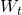• a percentage drift• a percentage volatilityThis then gives

(2)whereis a standard brownian motion andthe initial value. By definition (3) is a geometric brownian motion since it solves the stochastic differential equation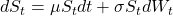. Assuming a geometric brownian motion is also the foundation of the famous black-scholes-model. Thusis log normally distributed with

(3)To answer our initial question we use a commonly known result based on the Girsanov theorem:

Proposition 1 (proof as Proposition 3.2)
Letbe a brownian motion with drift, let,then

(4)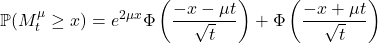hereis CDF of the standard normal distribution.

When we apply (4) to the geometric brownian motion withwe get

(5)where.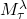is thus a brownian motion with drift, in addition using a scaling with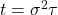of the underlying brownian motion, this lead to

(6)Accordingly, by symmetrie we get for(7)The equations above thus tell us how likely it is to sell/buy a stock within a time interval of length. To answer our initial question (1) we derive the expectation, to get the best buy and sell limits, with

(8)## EstimatingandSinceandare unknown we have to estimate them from observations. Let, which obviously follows a normal distribution with meanand variance. Thusand.
Stock prices are usually not observed as a continuous process, but instead oftentimes on a daily or minutely basis. In fact stock prices are never continuous because price fluctuations are measured using ticks. This is one reason, among others, why a brownian motion can only be considered as an approximation of the true behaviour of a stock price. If we assumediscrete observationswith starting pointand endpointwith an equidistant time gap ofwe can derive unbiased estimators

(9)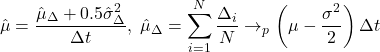while

(10)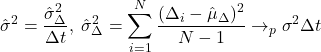further we can verify that the estimators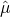,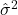are consistent with

(11)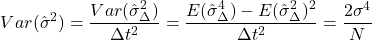(12)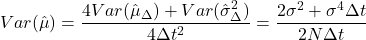## Real Data Example

We use the above described method write a small python script that will estimateandfor a particular stock and then derive the optimal buy or sell limit dependent on.

Try it yourself with my online notebook:

Figure 1: A picture of the python program. Beside the estimates forandas well asalso isshown. The blue line shows the share price of the DAX between 1988 and today.The orange line is the trend.

This site uses Akismet to reduce spam. Learn how your comment data is processed.